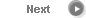#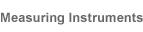# Precise Color Communication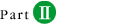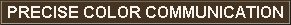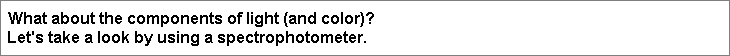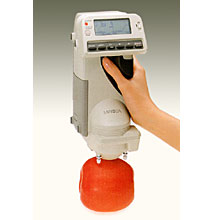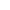An object absorbs part of the light from the light source and reflects the remaining light. This reflected light enters the human eye, and the resulting stimulation of the retina is recognized as the object's "color" by the brain. Each object absorbs and reflects light from different portions of the spectrum and in different amounts; these differences in absorptance and reflectance are what make the colors of different objects different.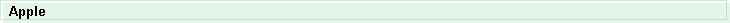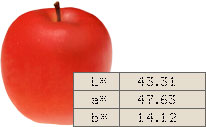If we measure an apple, we obtain the spectral graph shown in Figure 17a. If we look at this graph, we see that in the red wavelength region the reflectance (the amount of reflected light) is high, but in other wavelength regions the reflectance (the amount of reflected light) is low. Figure 17b shows that the apple reflects light in the orange and red wavelength regions and absorbs light in the green, blue, indigo, and violet wavelength regions. In this way, by taking a measurement with a spectrophotometer and displaying the results on a spectral graph, we can see the nature of the apple's color. Each of the multiple sensors (40 in the Konica Minolta Spectrophotometer CM-2002) of a spectrophotometer measures light in a strictly defined wavelength region of the visible light wavelength range. Because of this, the spectrophotometer can measure differences in the elements of color which are not noticeable for the human eye.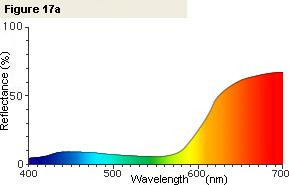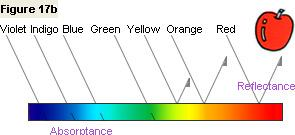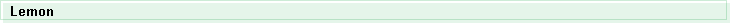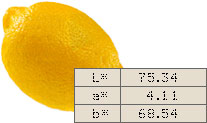If we measure a lemon, we obtain the spectral graph shown in Figure 18a. If we look at this graph, we see that in the red and yellow wavelength regions the reflectance (the amount of reflected light) is high, but in the indigo and violet wavelength regions the reflectance (the amount of reflected light) is low. Figure 18b shows that the lemon reflects light in the green, yellow, and red wavelength regions and absorbs light in the indigo and violet wavelength regions. This is the nature of the lemon's color. Such high accuracy is not possible with the human eye or even with the colorimeters discussed in Part I ; it is only possible with a spectrophotometer.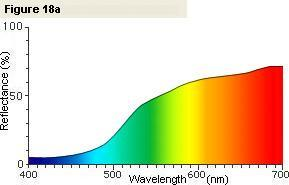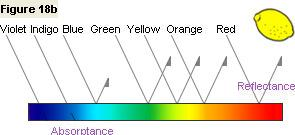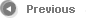4/9